# Unofficial Deep Learning Lecture 2 Notes

Hi All,

Here’s my lecture 2 notes. Hope it’s useful.

## Part 1: Overview of Dogs vs. Cats Image Recognition

Resources mainly from lesson 1 from the GitHub repository.

``````# Put these at the top of every notebook, to get automatic reloading and inline plotting
%matplotlib inline
``````
``````import torch
from fastai.imports import *
``````
``````from fastai.transforms import *
from fastai.conv_learner import *
from fastai.model import *
from fastai.dataset import *
from fastai.sgdr import *
from fastai.plots import *
``````

### Opening

#### Recap: we made a simple classifier last week with dogs and cats.

How do we tune these neural networks? Learning rate. Practice. Epoch number.

#### Sample Code

``````arch=resnet34
data = ImageClassifierData.from_paths(PATH, tfms=tfms_from_model(arch, sz))
learn = ConvLearner.pretrained(arch, data, precompute=True)
learn.fit(0.01, 3)
``````

#### Output

``````A Jupyter Widget
[ 0.       0.04726  0.02807  0.99121]
[ 1.       0.04413  0.02372  0.99072]
[ 2.       0.03454  0.02609  0.9917 ]
``````
``````PATH = "/home/paperspace/Desktop/data/dogscats/"
sz=224
arch=resnet34
``````
``````data = ImageClassifierData.from_paths(PATH, tfms=tfms_from_model(arch, sz))
``````
``````learn = ConvLearner.pretrained(arch, data, precompute=True)
learn.fit(0.01, 3)
``````
``````A Jupyter Widget
[ 0.       0.04247  0.02314  0.9917 ]
[ 1.       0.03443  0.02482  0.98877]
[ 2.       0.03072  0.02676  0.98975]
``````

## Choosing a learning rate

The thing that most determines how we are going to zoom in or hone in on the solution. Where is the “minimum point”. How do you find the minimum point?

If i was a computer algorithm, how do i found the minimum. The learning rate is how big of a jump that we will advance (the size of the arrow in the image below).

### If your learning rate is too high:### Learning rate finder

``````learn = ConvLearner.pretrained(arch, data, precompute=True)
``````

This is a custom function.

### The ConvLearner Class

`````` def __init__(self, data, models, precompute=False, **kwargs):
self.precompute = False
super().__init__(data, models, **kwargs)
self.crit = F.binary_cross_entropy if data.is_multi else F.nll_loss
if data.is_reg: self.crit = F.l1_loss
elif self.metrics is None:
self.metrics = [accuracy_multi] if self.data.is_multi else [accuracy]
if precompute: self.save_fc1()
self.freeze()
self.precompute = precompute

@classmethod
def pretrained(self, f, data, ps=None, xtra_fc=None, xtra_cut=0, **kwargs):
models = ConvnetBuilder(f, data.c, data.is_multi, data.is_reg, ps=ps, xtra_fc=xtra_fc, xtra_cut=xtra_cut)
return self(data, models, **kwargs)

@property
def model(self): return self.models.fc_model if self.precompute else self.models.model

@property
def data(self): return self.fc_data if self.precompute else self.data_

def create_empty_bcolz(self, n, name):
return bcolz.carray(np.zeros((0,n), np.float32), chunklen=1, mode='w', rootdir=name)

def set_data(self, data):
super().set_data(data)
self.save_fc1()
self.freeze()

def get_layer_groups(self):
return self.models.get_layer_groups(self.precompute)

def get_activations(self, force=False):
tmpl = f'_{self.models.name}_{self.data.sz}.bc'
# TODO: Somehow check that directory names haven't changed (e.g. added test set)
names = [os.path.join(self.tmp_path, p+tmpl) for p in ('x_act', 'x_act_val', 'x_act_test')]
if os.path.exists(names) and not force:
self.activations = [bcolz.open(p) for p in names]
else:
self.activations = [self.create_empty_bcolz(self.models.nf,n) for n in names]

def save_fc1(self):
self.get_activations()
act, val_act, test_act = self.activations

if len(self.activations)==0:
m=self.models.top_model
predict_to_bcolz(m, self.data.fix_dl, act)
predict_to_bcolz(m, self.data.val_dl, val_act)
if self.data.test_dl: predict_to_bcolz(m, self.data.test_dl, test_act)

self.fc_data = ImageClassifierData.from_arrays(self.data.path,
(act, self.data.trn_y), (val_act, self.data.val_y), self.data.bs, classes=self.data.classes,
test = test_act if self.data.test_dl else None, num_workers=8)

def freeze(self): self.freeze_to(-self.models.n_fc)
``````

## What the Fastai library does:

• tries to find the fastest way to converge to a solution.

#### Best thing to do for your model is get more data:

Problem: models will eventually start memorizing answers, this is called overfitting. Ideally more data will prevent this occurrence. There’s other techniques to assist with gathering more data.

#### Data augmentation (from lesson 1)

If you try training for more epochs, you’ll notice that we start to overfit, which means that our model is learning to recognize the specific images in the training set, rather than generalizing such that we also get good results on the validation set. One way to fix this is to effectively create more data, through data augmentation. This refers to randomly changing the images in ways that shouldn’t impact their interpretation, such as horizontal flipping, zooming, and rotating.

We can do this by passing `aug_tfms` (augmentation transforms) to `tfms_from_model`, with a list of functions to apply that randomly change the image however we wish. For photos that are largely taken from the side (e.g. most photos of dogs and cats, as opposed to photos taken from the top down, such as satellite imagery) we can use the pre-defined list of functions `transforms_side_on`. We can also specify random zooming of images up to specified scale by adding the `max_zoom` parameter.

#### Transformations library

We can use the available options to change the zoom, rotate and shift variations.

``````tfms = tfms_from_model(resnet34,
sz,
aug_tfms=transforms_side_on,
max_zoom=1.1)
``````
``````def get_augs():
data = ImageClassifierData.from_paths(PATH, bs=2, tfms=tfms, num_workers=1)
x,_ = next(iter(data.aug_dl))
return data.trn_ds.denorm(x)
``````
``````ims = np.stack([get_augs() for i in range(6)])
``````
``````plots(ims, rows=2)
``````

#### Other Options

``````transforms_side_on
transforms_top_down
``````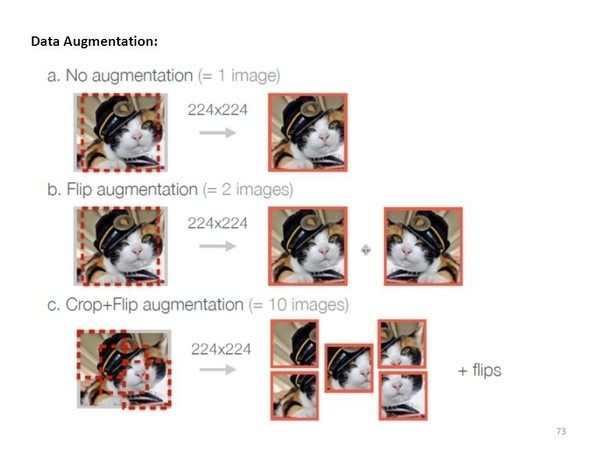### Why do we use the learning rate that isn’t the lowest point?

Each time we iterate, we will double the learning rate. The purpose of this to find what learning rate is helping use to decrease quickly. The learning rate is going too high.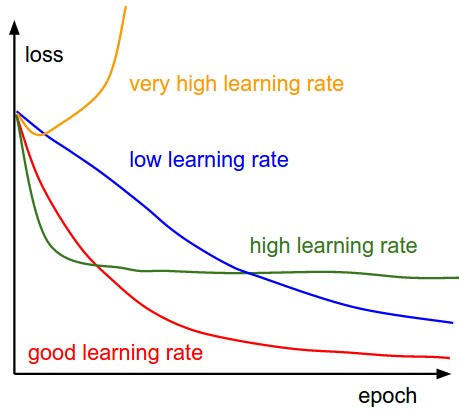### Comment: this augmentation won’t doing anything because of precompute

Note, we are using a pretrained network. We can take the 2nd last layer and save those activations. There is this level of “dog space” “eyeballs” etc. We save these and call these pre-computed activations.

#### Making a new classifier from precompute

We can quickly train a simple linear model based on these saved precomputed numbers. So the first time you run a model, it will take some time to calculate and compile. Then afterwards, it will train much faster.

``````data = ImageClassifierData.from_paths(PATH, tfms=tfms)
learn = ConvLearner.pretrained(arch, data, precompute=True)
learn.fit(1e-2, 1)
``````
``````[ 0.       0.04783  0.02601  0.99023]
``````

Since we have precomputed the different cat pictures don’t help. So we will turn it off.

By default when we create a learner, it sets all but the last layer to frozen. That means that it’s still only updating the weights in the last layer when we call fit.

``````learn.precompute=False
learn.fit(1e-2, 3, cycle_len=1)
``````
``````[ 0.       0.0472   0.0243   0.99121]
[ 1.       0.04335  0.02358  0.99072]
[ 2.       0.04403  0.0229   0.99219]
``````

## Cycle Length = 1

As we get closer, we may want to decrease the learning rate to get a more precise. Also known as annealing.

#### Most common annealing:

Pick a rate, then drop it 10x, then drop again. Stepwise, very manual. A simpler approach is to choose a functional form such as a line. Turns out that half a cosine curve works out well.

##### What do you do when you have more than one minima?

Sometimes one minima will be better than others (based on how well it generalizes). So sharply changing the learning rate has the idea that if we suddenly jump up the learning rate, we will get out of “narrow” minimum and find the most “generalized” minimum.

#### Note that annealing is not necessarily the same as restarts

We are not starting from scratch each time, but we are ‘jumping’ a bit to ensure we are in the best minima.

##### From the lesson 2 notebook:

What is that `cycle_len` parameter? What we’ve done here is used a technique called stochastic gradient descent with restarts (SGDR), a variant of learning rate annealing, which gradually decreases the learning rate as training progresses. This is helpful because as we get closer to the optimal weights, we want to take smaller steps.

However, we may find ourselves in a part of the weight space that isn’t very resilient - that is, small changes to the weights may result in big changes to the loss. We want to encourage our model to find parts of the weight space that are both accurate and stable. Therefore, from time to time we increase the learning rate (this is the ‘restarts’ in ‘SGDR’), which will force the model to jump to a different part of the weight space if the current area is “spiky”. Here’s a picture of how that might look if we reset the learning rates 3 times (in this paper they call it a “cyclic LR schedule”):

(From the paper Snapshot Ensembles).

The number of epochs between resetting the learning rate is set by `cycle_len`, and the number of times this happens is referred to as the number of cycles, and is what we’re actually passing as the 2nd parameter to `fit()`. So here’s what our actual learning rates looked like:

``````learn.sched.plot_lr()
``````

#### Good Tip: Save your weights as you go!

``````learn.save('224_lastlayer')
``````

### Fine-tuning and differential learning rate annealing

Now that we have a good final layer trained, we can try fine-tuning the other layers. To tell the learner that we want to unfreeze the remaining layers, just call (surprise surprise!) `unfreeze()`.

``````learn.unfreeze()
``````

#### In general you can only freeze layer from ‘n’ and on

Note that the other layers have already been trained to recognize ImageNet photos (whereas our final layers where randomly initialized), so we want to be careful of not destroying the carefully tuned weights that are already there.

Generally speaking, the earlier layers (as we’ve seen) have more general-purpose features. Therefore we would expect them to need less fine-tuning for new datasets. For this reason we will use different learning rates for different layers: the first few layers will be at `1e-4`, the middle layers at `1e-3`, and our Fully Connected (FC) layers we’ll leave at `1e-2` as before. We refer to this as differential learning rates, although there’s no standard name for this technique in the literature that we’re aware of.

### Specifying learning rates

We are going to specify ‘differential learning rates’ for different layers. We are grouping the blocks (ResNet blocks) in different areas and assigning different learning rates.

Reminder: we unfroze the layers and now we are retraining the whole set. The learning rate is smaller for early layers and making them larger for the ones farther away.

``````lr=np.array([1e-4,1e-3,1e-2])
``````
``````# 3 is the number of cycles
# 3 cycles of 2 epochs
learn.fit(lr, 3, cycle_len=1, cycle_mult=2)
``````
``````[ 0.       0.04913  0.02252  0.99268]
[ 1.       0.04842  0.02123  0.99219]
[ 2.       0.03309  0.02412  0.99121]
[ 3.       0.03528  0.02148  0.99072]
[ 4.       0.02364  0.02106  0.99023]
[ 5.       0.01987  0.01931  0.9917 ]
[ 6.       0.01994  0.02058  0.99121]
``````

#### `cycle_mult` parameter

It doubles the length of the cycle after each cycle.

Another trick we’ve used here is adding the `cycle_mult` parameter. Take a look at the following chart, and see if you can figure out what the parameter is doing:

``````learn.sched.plot_lr()
``````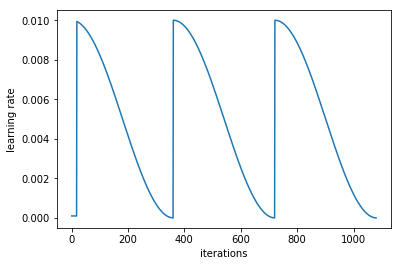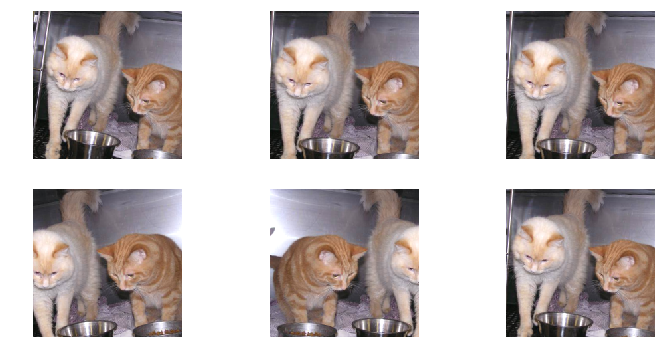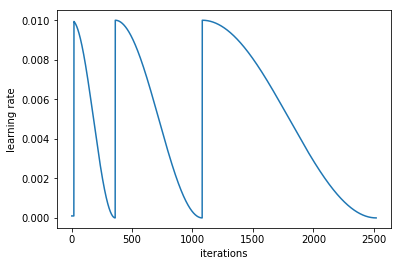#### At this point, we are going to look back at incorrect pictures

We are going to do. Use test time augmentation we are going to take 4 random data augmentation. Move them around and flip and mix with the prediction. We are going to average all the predictions of the original + permutation. Ideally the rotating + zoom will get it in the right orientation.

#### Test-Time-Augmentation (TTA)

TTA makes predictions not only on the originals but also on the random augmented generated.

``````log_preds,y = learn.TTA()
accuracy(log_preds,y)
``````
``````0.99199999999999999
``````

# Overview of the Steps

1. Enable data augmentation, and precompute=True
2. Use `lr_find()` to find highest learning rate where loss is still clearly improving
3. Train last layer from precomputed activations for 1-2 epochs
4. Train last layer with data augmentation (i.e. precompute=False) for 2-3 epochs with `cycle_len=1`
5. Unfreeze all layers
6. Set earlier layers to 3x-10x lower learning rate than next higher layer
7. Use `lr_find()` again
8. Train full network with `cycle_mult=2` until over-fitting

# Dog Breeds

``````PATH = '/home/paperspace/Desktop/data/dogbreeds/'
``````
``````# Put these at the top of every notebook, to get automatic reloading and inline plotting
%matplotlib inline
``````
``````import torch
from fastai.imports import *
``````
``````from fastai.transforms import *
from fastai.conv_learner import *
from fastai.model import *
from fastai.dataset import *
from fastai.sgdr import *
from fastai.plots import *
``````
``````sz=224
arch=resnet34
bs=24
``````
``````label_csv = f'{PATH}labels.csv'

#list of rows, minus 1, nubmer of rows in CSV, number of imgs
n = len(list(open(label_csv)))-1

# get crossvalidation indexes custom FASTAI
val_idxs = get_cv_idxs(n)
``````
``````n
``````
``````10222
``````
``````val_idxs
``````
``````array([3694, 1573, 6281, ..., 5734, 5191, 5390])
``````

#### Will get 20% of the data will be in the validation set

``````??get_cv_idxs
``````
``````def get_cv_idxs(n, cv_idx=4, val_pct=0.2, seed=42):
np.random.seed(seed)
n_val = int(val_pct*n)
idx_start = cv_idx*n_val
idxs = np.random.permutation(n)
return idxs[idx_start:idx_start+n_val]
``````

# Initial Exploration

``````!ls {PATH}
``````
``````labels.csv	sample_submission.csv.zip  test.zip  train
labels.csv.zip	test			   tmp	     train.zip
``````
``````label_df = pd.read_csv(label_csv)
``````
``````label_df.head()
``````
id breed
0 000bec180eb18c7604dcecc8fe0dba07 boston_bull
1 001513dfcb2ffafc82cccf4d8bbaba97 dingo
2 001cdf01b096e06d78e9e5112d419397 pekinese
3 00214f311d5d2247d5dfe4fe24b2303d bluetick
4 0021f9ceb3235effd7fcde7f7538ed62 golden_retriever
``````label_df.pivot_table(index='breed', aggfunc=len).sort_values('id', ascending=False)[:10]
``````

id
breed
scottish_deerhound 126
maltese_dog 117
afghan_hound 116
entlebucher 115
bernese_mountain_dog 114
shih-tzu 112
great_pyrenees 111
pomeranian 111
basenji 110
samoyed 109
``````tfms = tfms_from_model(arch, sz, aug_tfms=transforms_side_on, max_zoom=1.1)
data = ImageClassifierData.from_csv(PATH, 'train' ,f'{PATH}labels.csv', test_name='test', val_idxs=val_idxs, suffix='.jpg', tfms=tfms, bs=bs)
``````
``````fn = PATH + data.trn_ds.fnames; fn
``````
``````'/home/paperspace/Desktop/data/dogbreeds/train/000bec180eb18c7604dcecc8fe0dba07.jpg'
``````
``````img = PIL.Image.open(fn); img
````````````img.size
``````
``````(500, 375)
``````

## How big are the images?

Most ImageNet models are trained on 224 x 224 or 299 x 299. Lets make a dictionary comprehension to store all the names of the files to the size of the files. This will be important for memory and size consideration.

``````size_d = {k: PIL.Image.open(PATH+k).size for k in data.trn_ds.fnames}
``````
``````row_sz, col_sz = list(zip(*size_d.values()))
``````
``````row_sz = np.array(row_sz); col_sz=np.array(col_sz)
``````
``````row_sz[:5]
``````
``````array([500, 500, 400, 500, 231])
``````

### Let’s look at the distribution of the Image Sizes (rows first)

Most of them are under 1000, so we will use NumPy to filter.

``````plt.hist(row_sz)
``````
``````(array([ 3014.,  5029.,    91.,    12.,     8.,     3.,    17.,     1.,     1.,     2.]),
array([   97. ,   413.7,   730.4,  1047.1,  1363.8,  1680.5,  1997.2,  2313.9,  2630.6,  2947.3,  3264. ]),
<a list of 10 Patch objects>)
``````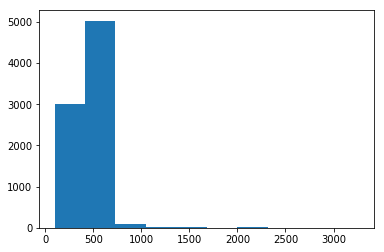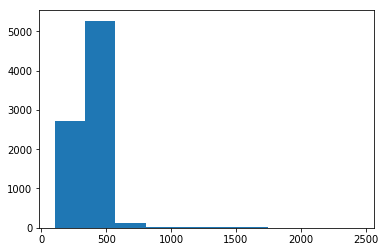``````plt.hist(row_sz[row_sz<1000])
``````
``````(array([  148.,   600.,  1307.,  1205.,  4581.,   122.,    78.,    62.,    15.,     7.]),
array([  97. ,  186.3,  275.6,  364.9,  454.2,  543.5,  632.8,  722.1,  811.4,  900.7,  990. ]),
<a list of 10 Patch objects>)
``````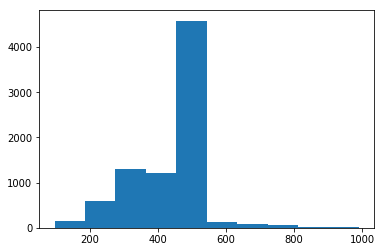### Let’s look at the distribution of the Image Sizes (cols)

``````plt.hist(col_sz)
``````
``````(array([ 2713.,  5267.,   131.,    21.,    15.,     8.,    17.,     4.,     0.,     2.]),
array([  102. ,   336.6,   571.2,   805.8,  1040.4,  1275. ,  1509.6,  1744.2,  1978.8,  2213.4,  2448. ]),
<a list of 10 Patch objects>)
````````````plt.hist(col_sz[col_sz<1000])
``````
``````(array([  243.,   721.,  2218.,  2940.,  1837.,    95.,    29.,    29.,     8.,     8.]),
array([ 102. ,  190.2,  278.4,  366.6,  454.8,  543. ,  631.2,  719.4,  807.6,  895.8,  984. ]),
<a list of 10 Patch objects>)
``````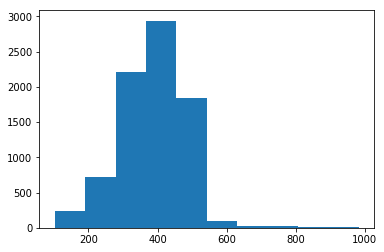### Let’s look at the classes

``````len(data.trn_ds), len(data.test_ds)
``````
``````(8178, 10357)
``````
``````len(data.classes), data.classes[:5]
``````
``````(120,
['affenpinscher',
'afghan_hound',
'african_hunting_dog',
'airedale',
'american_staffordshire_terrier'])
``````

# Initial Model

``````def get_data(sz, bs):
tfms = tfms_from_model(arch, sz, aug_tfms=transforms_side_on, max_zoom=1.1)
data = ImageClassifierData.from_csv(PATH, 'train' ,f'{PATH}labels.csv', test_name='test', val_idxs=val_idxs, suffix='.jpg', tfms=tfms, bs=bs)
return data if sz > 300 else data.resize(340, 'tmp')
``````

## Precompute

``````data = get_data(sz,bs)
``````

### Used ResNet34 since ResNext didn’t load for some errors

``````learn = ConvLearner.pretrained(arch,data, precompute=True, ps=0.5)
``````
``````  0%|===================================>| 0/341 [00:00<?, ?it/s]
``````
``````learn.fit(1e-2,2)
``````

## Do a few more cycles, more epochs

Epoch - 1 pass through the data.
Cycle - is how many epoches in a full cycle.

#### offline - tried to find the learning rate

``````learn.precompute=False
learn.fit(1e-2, 5, cycle_len =1)
``````

### Can continue training on larger images after starting on smaller images

Started with 224 x 224, and continuing with 299 x 299. Will start small then move to larger general images to limit the overfitting.

#### Some addition trial and error training

``````learn.set_data(get_data(299,bs))
learn.fit(1e-2,3,cycle_len=1)
learn.fit(1e-2,3 cycle_len=1, cycle_mult=2)
``````

## Scoring

``````log_preds,y = learn.TTA()
probs = np.exp(log_preds)
accuracy(log_preds,y), metrics.log_loss(y, probs)
``````
84 Likes

6 Likes

@timlee, Nice work ! Thanks for the notes. Appreciate it !

1 Like

Great Work! Could you kindly share the raw markdown?2 Likes

yes I can share the raw MD if needed. Let me know which format is preferred, Jnotebook or MD

thank you @ecdridAlso you can convert your notebooks to `.md` just use this…

``````jupyter nbconvert --to <output format> <input notebook>

``````

Possible Formats

• HTML
• LaTeX
• PDF
• Reveal JS
• Markdown (md)
1 Like

# Lesson 2 Notes

## Overview

Lesson 2 consists of a review of Lesson 1, digging into the learning rate, and data augmentation. It walks through using these techniques to present a common framework for making a good classifier, and then applies it to a Kaggle competition on dogs and cats.

This lesson covers topics in cycles of increasing depth as opposed to linearly and some important information comes from the question and answer sessions. I use timestamps to the YouTube video; (10:12) hints related material starts at ten minutes and twelve seconds.

## Review

(0:00) In the first section, we used four lines of library code to create and train an image classifier. The dogscats dataset split validation training data into `valid/` and `train/` directories, each having subdirectories for the labels `dogs` and`cats`, so `data/dogscats/valid/dogs/dogs.3085.jpg` is the dogscats data set, validation data, labelled dogs, in a jpg file named `dogs.3085.jpg`. Most people doing homework used the same directory label for a different data set. (1:18:00) In contrast, a data layout using labels in a csv file and a random validation split is used in the Kaggle Competition section.

To review, a Data set is the entire data set, usually in one subdirectory under data/. A Label is
the ‘answer’, e.g., ‘dog’ or ‘cat’, that is the correct classification for each image. The Training Set data trains the neural net, mapping images to a label and adjusting the weights and biases of its nodes until the mapping generates the same labels as given. The Validation Set is used solely to evaluate the neural net training. The net (or model) looks at the image, chooses a classification, and marks it as correct if the most likely classification matches the given label. The Model is another name for the trained neural net, also called a classifier when the job is to classify. It consists of multiple layers of neurons (nodes) each with a weight (multiplied) and bias (added) connected into a particular architecture.

### Our Four Line Image Classifier

(5:00) The above sample code adjusts the Learning Rate, an adjustment to how fast the neural network adjusts weights. Setting a good rate will be the bulk of this lecture. Each Epoch is a pass through the data, running many mini-batches each updating weights, and then calculating the loss and accuracy of the new weights against the validation set. The Loss averages the ‘badness’ of each guess: “very sure its a cat” would give a loss near one if the image were a dog. The Accuracy is a simple ratio of the correctly guessed classifications over the number of samples, where the guessed classification is whichever one the model thinks is most likely, regardless of certainty. (1:12:00) You might also use a Confusion Matrix image to show true versus predicted labels.

## Learning Rate

(4:50) The learning rate is an adjustment of how fast the neural network adjusts weights during training, or fast you try to hone in a solution. It is a Hyperparameter, meaning a tunable parameter of your machine learning engine. The FastAi Library picks reasonable values for dozens of hyper-parameters and reasonable choices for algorithms. You will learn when to override defaults throughout the course, and the library provides an estimator rather than a specific value for the learning rate. The learning rate is the most important hyperparameter.

You can think of learning rate as length of the vector used in a step of gradient descent. If it is too low, the model will take too long to find the minimum and may get ‘stuck’ in a small local minima. If it is too high, it will jump around and follow a gradient to its nadir (lowest point). If you ever find your loss values going to infinity, your learning rate is too high.

There are three major methods presented: an algorithm for finding a good constant learning rate, simple Learning Rate Annealing where you decrease the learning rate as you train, and annealing with restarts where the learning rate decreases but gets reset after some number of epochs. There are also Differential Learning Rates which apply different rates to different layers of the model. All of these start by finding a good constant learning rate.

### Constant Learning Rate with lr_find()

(7:30) The FastAI Library provides a Learning Rate Finder, or `learn.lr_find()`:

1. starting in random place with a small learning rate.
2. on each minibatch, run a step, calculate the loss, and then multiplicitively increase the learning rate
3. ignore the `learn.lr_find()` output; look at `learn.sched.plot_lr()` and `learn.sched.plot()`.
4. best learning rate, is one order (10x) less than rate with minimum loss. In this case, 10e-2 (=0.01)

Three visualizations of this algorithm while finding the same learning rate:

### Learning Rate Annealing and SGDR

(34:00) Learning Rate Annealing is decreasing the learning rate as you train, hoping to find the nadir of the cost vs iteration function. It is a very common and popular technique. Three simple annealing methods start the learning rate at a constant (as found above) and gradually decrease the learning rate each mini-batch: Step-wise Annealing uses discrete decreases, sometimes in a manual or hacky way; Linear Annealing decreases learning rate a set amount per iteration; and Cosine Annealing uses the cosine curve from 0 to 90 degrees to reduce slowly then quickly then slowly again. A more complicated method would good results is Stochastic Gradient Decent With Restarts (SGDR) which periodically resets a simple (usually cosine) linear annealing curve every one or more epochs. This hopes to settle in “broad valleys” corresponding to good solutions that aren’t too specific to the specific training images. Use FastAI Library’s SGDR by adding the `cycle_len=1` parameter to `learn.fit()`. (53:00) A final tweak on SGDR is to add the `cycle_mult=2` parameter to continually increase the number of epochs between restarting the linear annealing curve.

These advanced SGDR routines work better than the approaches of a Grid Search (systematic search for good hyperparameter values) for a learning rate or Ensemble (run multiple models and take best result) of different starting points.

Compare the different learning rates over time with different methods.

Consider how SGDR finds the general area of an annoying curve but simple annealing does not.

(46:30) After unfreezing the Early, Middle, and Later layers, we allow our training data to
adjust the weights of these layers, see About the Model. You can use Differential Learning Rates where different layers of the neural network have different training rates to tune the early layers less than middle layers less than later layers. To do this fine-tuning, use `learn.unfreeze()` then pass it to `learn.fit()`. (1:15:45) Usually use ratios of about 10x for regular images or 3x for medical or satellite images.

## Data Augmentation

(15:00) Getting enough labeled data is always a problem. Without enough data, you get Overfitting where the network too closely memorizes artifacts of input data at expense of the actual meaning. Overfitting manifests as much lower training loss (more confidence) on your training set than your validation set and decreasing accuracy at later epochs. Getting more data is one solution.

Data Augmentation is creating additional synthetic data from existing labelled such that the bits change but not the meaning. This is done with transformation functions, e.g., “make new images by rotating the original image randomly between -5 degrees and +5 degrees”. The bits in each transformed image will be unique but the it will still be a cat. A typical set of transformations may include shifting, zooming, flipping, small rotations, or small brightness changes. A human chooses the set of augmentations that preserve meaning: faces flipped upside down are no longer normal faces. The FastAI Library lets you create a set of transforms (`tfms_from_model`) and pass them to the image classifier. At each minibatch during training, originals and random transformations will be used to train the model. (1:01:40) Padding and fixed sliding windows don’t work well and good transformations for non-image worlds are under-researched.

(54:30) You can also use Test Time Augmentation, which uses data augmentation at the testing time. Instead of classifying the validation image, classify the validation image and four random transformations of it and then take the average.

Activation of any one node in the network is a number corresponding with how much one set of inputs match what the node was trained towards. (44:00) (1:45:00) A model can be thought of as Early Layers, Middle Layers, Later Layers, and a Final Layer. The ResNet model we use is based on a network already trained (weighted and biased) on all images of ImageNet. The Early Layers activate strongly to gradients and edges; the Middle Layers activate strongly to corners, circles and loops; and Later layers activate strongly to eyes, pointed ears, centers of flowers, faces, etc. In the original ResNet, the Final Layer connected to end of the Later layers and classified into hundreds of labels. (1:39:40) For our Cats and Dogs Model, the Final Layer was replaced with two layers to classify only Dog and Cat labels.

We are usually training only the final layer. The other layers (early, middle, and later) are Frozen, fixed such that training data will not affect their values. For efficiency, we use Precomputed Activations where we cache the activation values of the last of the Later Layers for each training image instead of recalculating each image each time it is in a minibatch, for about a 10x speedup. (41:00) We use precomputed activations with
`ConvLearner.pretrained(arch, data, precompute=True)` and these are stored in a `dogscats/tmp` directory. The first execution on a new dataset takes extra time to build the cache, except for Crestle which takes extra time more often. If everything hangs, try deleting the `dogscats/tmp` directory to reset the caches.

We need to turn off precomputed activations (`learn.precompute = False`) to use Data Augmentation. We unfreeze (turn off frozen) to use Differential Learning Rates.

(1:05:30) The FastAI Library is open source on top of PyTorch, which is this year’s hot library. The FastAI Library makes PyTorch easier, but the concepts are the important part. In production, you might export the PyTorch models or recode in TensorFlow for use on phones.

## Kaggle Competition

(1:13:52) To summarize the first hour, there is a sequence that usually can create a really good model. Roughly, its find the learning rate, train the last layer for couple epochs, train again with data augmentation, then differential learning rates, find the learning rate again, and finally train with SGDR w/cycle_mult.

(1:17:00) We will do this for the Kaggle competition on identifying breeds of dogs. We download the data with Kaggle CLI, get it loaded, and (1:23:30) explore the image sizes in
the sample. (1:26:40) We choose 20% to be the validation set.

While we first check our model with tiny images, we train with reasonable size. (1:33:00) After initial
training, we increase our image size to prevent overfitting.

We get a pretty good result without too much work. (1:53:00) We can also do this on the Planets competition.

(1:48:25) FYI, do even better on DogsCats using ResNext model instead!

While no homework is listed, there are a number of Kaggle competitions to try.

Shameless Plug: I’m leaving PARC at the end of January, 2018 and am looking for a fun start-up in Silicon Valley. charles.merriam@gmail.com

31 Likes

@CharlesMerriam that’s great! Would you be interested in contributing to our documentation project (with full credit of course)? It uses markdown, so we could potentially use your notes with just some minimal editing…Absolutely! I’ll also post the full sized slides (ppt) when I’m back at the right machine on Monday.

Let me know if you need any other action on my part; this parsed as just asking my permission.

No action required on your part - but more an optional thing that if you feel like there’s any edits you’d want to make to make your notes more widely useful, please do!@jeremy Could you turn this into wiki so others can contribute or edit? Thanks.

Done.

Hi everyone. I have done a quick proof reading and fixed, cleanup, corrected typos, reformatted style, etc. to ALL the lesson/lecture notes for version 2018 (aka part 1 v2) of this course. I hope the lecture notes are more pleasure to read now with those fixes in.

7 Likes

My hero!4 Likes

it provides better learning experience

I have been trying to understand this for a few time. I have watched lesson 2 in this regard.
Why is there the need for

precompute=True

in the above step for data augmentation step 4.

Please correct me if I am wrong. What I understand is: precompute is helping us getting the output(of the initial pretrained layers) for the same input again as no changes in all epochs. If we see an image we compute its output, save it, we see it again so we retreive that value as those initial layers are freezed so no need to compute again.

As we did data augmentation in the beginning so we already have all the data set with all the transformations. So when we did the step 3 of training the last layer we already have the values stored(for all the images including original as well as augmented ones), so why do `precompute=False` when using `cycle_len=1` in step 4. And how can we say we are using data augmentation specifically in this case as the dataset is same since we have applied the transformations. If we set precompute to True in step 4 can’t we increase the efficiency?

2 Likes

Great work @timlee.

I noticed a typo here:

learn.set_data(get_data(299,bs))
learn.fit(1e-2,3,cycle_len=1)
learn.fit(1e-2,3 cycle_len=1, cycle_mult=2)

There should be a comma “,” on the last line after the “3” and before “cycle_len=1”

Thank you.
Lou

1 Like

in the get_data function , why are we resizing the using data.resize(340 , ‘tmp’) , instead it should be resized to using following : data.resize(sz , 'tmp)

Also why are we passing sz argument in tfms_from_model function.